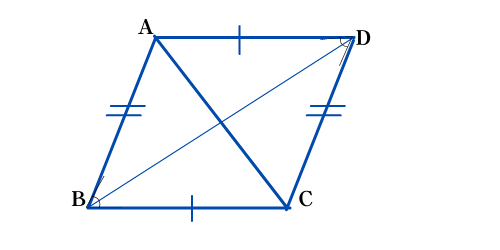# Parallelogram

In this article you will learn the concept of parallelogram, types of parallelogram and properties of parallelogram.

Before learning parallelogram, let’s understand the term “parallel”.

What is parallel?
Parallel lines are lines in a plane that never intersect or touch each other at any point. They are always the same distance apart and go side by side without ever touching each other.## What is parallelogram?

A parallelogram is a special type of quadrilateral that has at least two sides that are parallel to each other.

## Six Properties of Parallelogram1. Opposite sides of Parallelogram are parallel to each other. Here, AB ∥ CD and AD ∥ BC

2. Opposite sides are congruent, means opposite sides are equal in length. AB = CD and AD = BC

3. Opposite angles are congruent, means opposite angles are equal in measure. angle B = angle D and angle A = angle C

4. Adjacent angles of a parallelogram are supplementary. Supplementary angles means two angles that add up to 180.
Angle A + Angle B = 180°

Angle B + Angle C = 180°

Angle C + Angle D = 180°

Angle D + Angle A = 180°

5. The diagonals of a parallelogram bisect each other. AC and BD bisect each other.

6. Each diagonal of a parallelogram separates it into two equal triangles. ACD ABC
Related Topic: Point Lines Line Segments and Rays

## 3 Types of Parallelogram

#### Rhombus• All sides are equal and opposite sides are parallel.
• Opposite angles are equal.
• The diagonals bisect each other at right angles.
• Here AB = BC = CD = DA. So ABCD is a rhombus.

#### Rectangle• All angles are 90 degrees.
• Opposite sides are parallel and equal.
• Diagonals are congruent.
• Each diagonal is angle bisector of opposite angle
• Here AB = DC and AD = BC
• Diagonal AC = Diagonal BD

#### Square• All sides are equal in length
• Each interior angle is right angle [90 degrees]
• Length of diagonals is equal
• Diagonals are perpendicular bisectors of each other
• Every square is a rectangle and a rhombus

## Area of ParallelogramArea = Base x Height [Area = b x h]
Example: A parallelogram has a base of 7 m and is 4 m high, what is its Area?

Area = 7 m x 4 m = 28m2

## Perimeter of a ParallelogramPerimeter = 2 x (base + side length) or Perimeter = 2(b+s)

Example:A parallelogram has a base of 15 cm and a side length of 5 cm, what is its Perimeter?

Perimeter = 2 × (15 cm + 5 cm) = 2 × 20 cm = 40 cm

Try Now!
Example 1: A parallelogram has a base of 21 cm and a side length of 7 cm, what is its Perimeter?

Example 2: A parallelogram has a base of 16 m and is 13 m high, what is its Area?

Translate »
Scroll to Top